# How to use OR function in Microsoft Excel

In this article, we will learn how to use OR function in Excel.

OR function in Excel runs a basic logical function to test two values or statements. OR function checks if any of the condition in the formula is True, returns True and it returns False only if all condition or statements are False.

Syntax:

=OR(logical1, [logical2], [logical3], …)

OR function can be used via many ways.
Let’s understand them one by one

Here we have some coloured balls and we need to test the condition to check whether the ball is valid or not.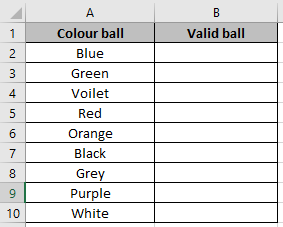Use the formula in B2 cell.

=OR(A2="Red",A2="White")

Explanation:
Function checks either Red ball or White ball is valid for the match to be played.
So it checks the cell A2 for the same.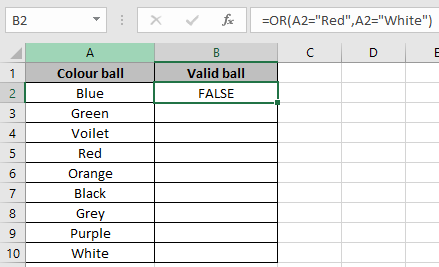As it returns False means it doesn’t have either Red or White.

Use the Ctrl + D option to check the cells corresponding to it.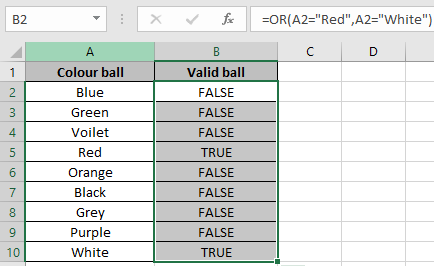As we can see in the above snapshot it returns True for the cells having Red or White coloured ball.

You can apply it to check on the range of cells to test whether any of the cell returns True or not.

Use the formula

=OR(A2:A10="Red",A2:A10="White")

Note: Don’t forget to use Ctrl + shift + Enter to get the result. Curly brackets will be visible in the function box (fx) of the cell.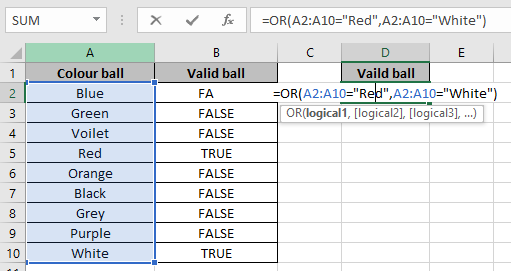Use Ctrl + Shift + Enter to see the result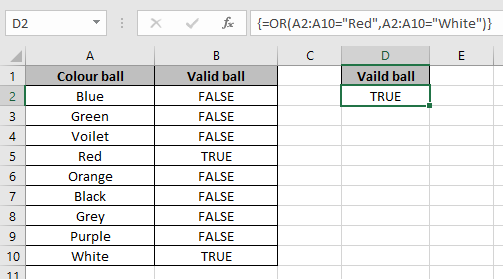It returns True. It means that A2:A10 range cells have either White or Red ball.

You can check less than or greater than condition on numbers. OR function can also be used along with many function like IF, AND and many more.

Hope you understood how to use OR function in Excel. Explore more articles on logic_test functions here. Feel free to state your queries in the comment box below.

Related Articles:

IF with OR Function in Excel

How to use the Excel AND Function

IF with AND Function in Excel

How to use the Excel TRUE Function

Excel NOT function

IF not this or that in Microsoft Excel

IF with AND and OR function in Excel

Popular Articles:

How to use the VLOOKUP Function in Excel

How to use the COUNTIF function in Excel 2016

How to Use SUMIF Function in Excel

## Users are saying about us...

1.I need a formula that will check each cell in a range and only apply highlight to the entire range IF none of the cells are blank. I am having a difficult time wrapping my brain around the verbiage and logic. I read something about needing to use ctlr + shift + enter - dynamic range?? Is this true? And what is it? I have been avoiding trying to learn VBA/Macros but fear that may be my only solution if there is not a way to highlight ranges based on my criteria. Can you help? I have tried COUNTA, IFS, and a little bit of macros without much success.

•You don't need to use array formula or VBA to do this work. Use this formula in conditional formatting,
=COUNTBLANK(range)=0

and add the highlights you want to do.

If any of the cells are blank in your range than the formula will be false and nothing will happen. Else the highlights will work.

Although it does not requires Array formula, it would be greate to learn what they are. It will help you: https://www.exceltip.com/excel-array-formulas/arrays-in-excel-formula.html

Terms and Conditions of use

The applications/code on this site are distributed as is and without warranties or liability. In no event shall the owner of the copyrights, or the authors of the applications/code be liable for any loss of profit, any problems or any damage resulting from the use or evaluation of the applications/code.